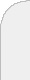inicioir al DIIbuscarpricing with markups in industries with increasing marginal costs We study a game that models a market in which heterogeneous producers of perfect substitutes make pricing decisions in a first stage, followed by consumers that select a producer that sells at lowest price. As opposed to Cournot or Bertrand competition, producers submit a price function to the market, which maps their production level to a price. Solutions of this type of models are normally referred to as supply function equilibria, and the most common application is in electricity markets. In our model, producers face increasing marginal production costs and, in addition, cost functions are proportional to each other, and their magnitudes depend on the efficiency of each particular producer. In this context, we prove necessary and sufficient conditions for the existence of equilibria in which producers use supply functions that replicate their cost structure. We then specialize the model to monomial cost functions with exponent equal to q>0, which allows us to reinterpret the simple supply functions as a markup charged on top of the production cost. We prove that an equilibrium for the markups exists if and only if the number of producers in the market is strictly larger than 1+q, and if an equilibrium exists, it is unique. The main result for monomial cost functions is to establish that the equilibrium is nearly efficient when the market is competitive. Here, an efficient assignment is one that minimizes the total production cost, ignoring payments because they are transfers within the system. The result holds because when there is enough competition, markups are bounded, thus preventing prices to be significantly distorted from costs. Finally, we focus on the case when unit costs are linear functions on the production quantities. This simplification allows us to refine the previous bound by establishing an almost tight bound on the worst-case inefficiency of an equilibrium. This bound is a subproduct of an algorithm that we design to find such equilibrium. The bound states that when there are two equally-efficient producers and possibly other less efficient ones, the production cost under an equilibrium is at most 50 percent worse than the optimal one, and the worst-case gap between the two assignments decreases rapidly as competition increases. For instance, for three similarly-efficient producers plus perhaps other less efficient ones, the inefficiency is below 6.2 percent.